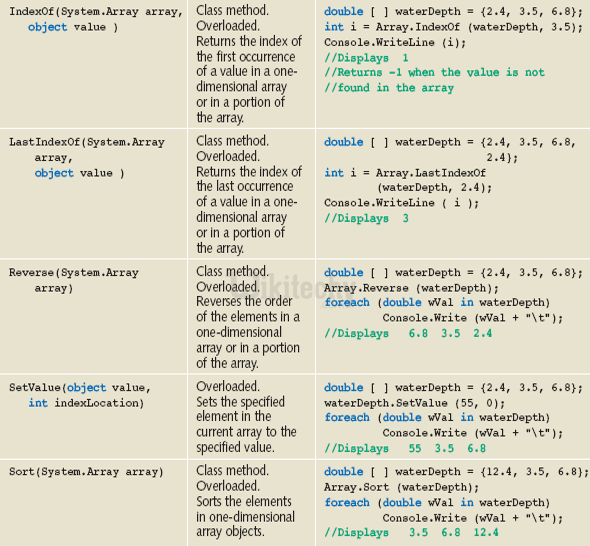# C# Array - c# - c# tutorial - c# net

## What is an array in C# ?

• In general, an arrays are collections of data.
• A scalar variable can hold the only one item at a time.
• Arrays can hold multiple items. These items are called elements of the array. Arrays store data of the same data type.
• Each element can be referred to by an index.
• Arrays are zero based. The index of the first element is zero. Arrays are reference types.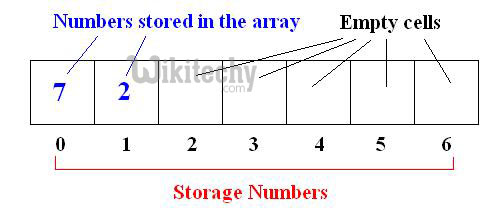## Syntax Explanation:

• datatype:
Datatype is used to specify the type of elements in the array. And [ ] specifies the rank of the array. The rank specifies the size of the array.
• arrayName:
ArrayName specifies the name of the array.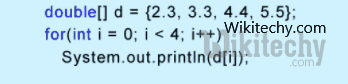c# Array

## C# Sample Code - C# Examples:1. int[] array = newint;We declare and initialize a numerical array. And we declare an array which contains 4 elements. All elements are integers.
2. We initialize the array with some data. This is assignment initialization. The indexes are in the square brackets. Array  is going to be the first element of the array.Array  is going to be the second element of the array, Array  is going to be the third element of the array, Array  is going to be the fourth element of the array.
3. Here for (int i = 0; i<5; i++) specifies integer i = 0, which means the expression, (I < 5), is true. Therefore, the statement is executed, and i gets incremented by 1 and becomes 4.
4. Here we can declare and initialize an array in one statementarray[i].

## Sample C# examples - Output :1. Here in this output the 1,2,3,4 will be printed until it reaches the less than "5" array elements.

## Array Vs ArrayList :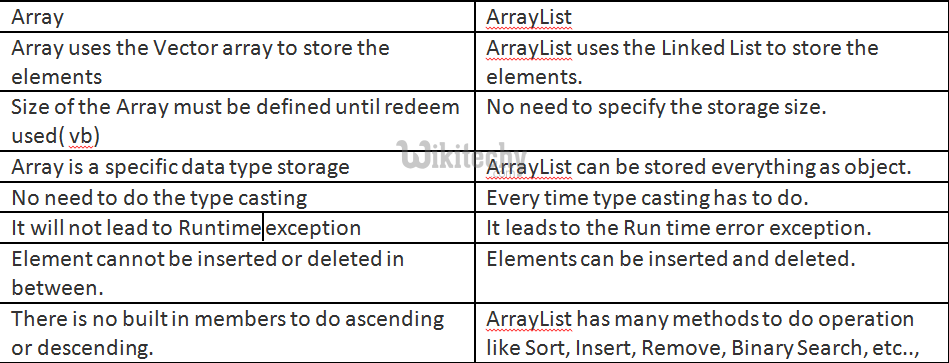learn c# tutorial - array vs arraylist csharp in c# Example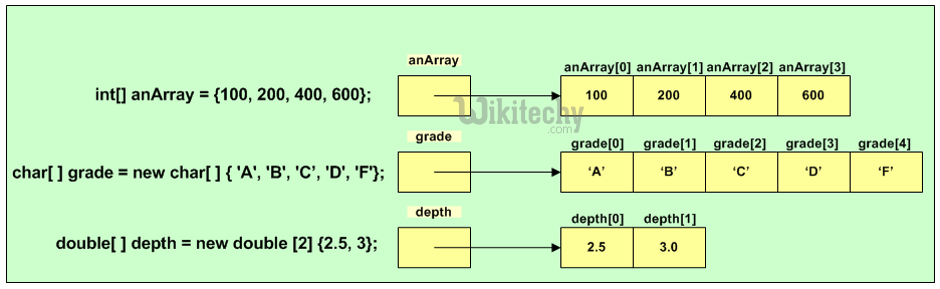learn csharp tutorial - c# collections - c# example programs